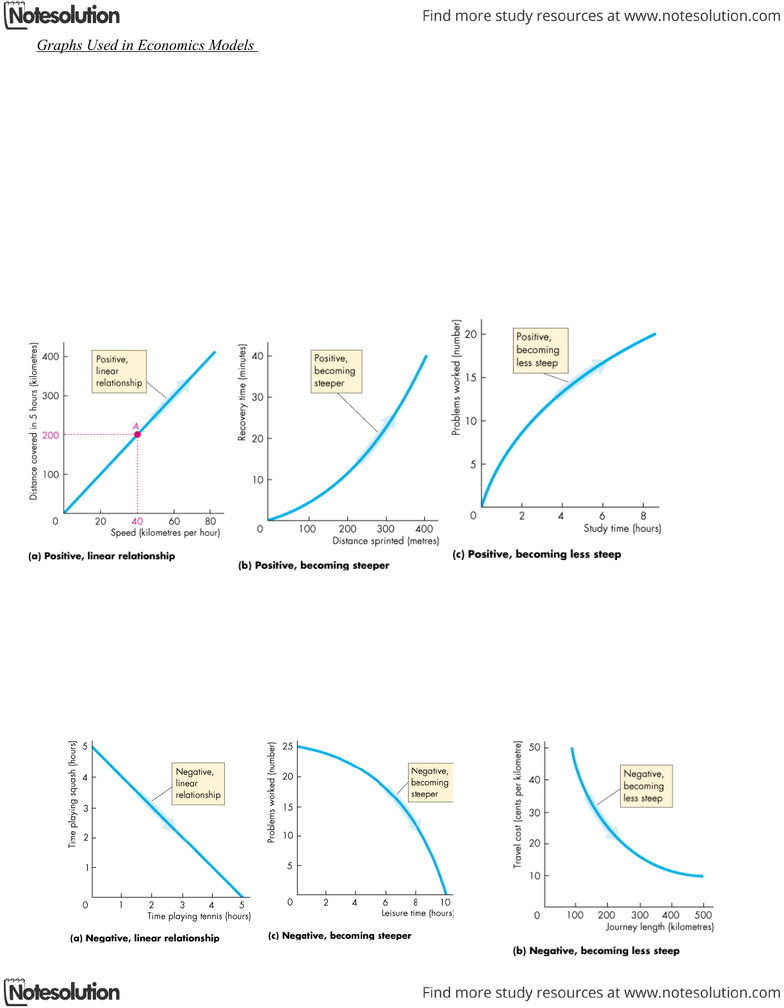# Graphs Used in Economics Models.doc

447 views1 pages
School
York University
Department
Economics
Course
ECON 1000
Page:
of 1Graphs Used in Economics Models
Graphs are used in economic models to show the relationship between variables.
The patterns to look for in graphs are the four cases in which
Variables move in the same direction.
Variables move in opposite directions.
Variables have a maximum or a minimum.
Variables are unrelated.
Variables That Move in the Same Direction
A relationship between two variables that move in the same direction is called a positive relationship or a direct
relationship.
A line that slopes upward shows a positive relationship.
A relationship shown by a straight line is called a linear relationship.
The three graphs on the next slide show positive relationships.
Variables That Move in Opposite Directions
A relationship between two variables that move in opposite directions is called a negative relationship or an
inverse relationship.
A line that slopes downward shows a negative relationship.
The three graphs on the next slide show negative relationships.

## Document Summary

Graphs are used in economic models to show the relationship between variables. The patterns to look for in graphs are the four cases in which. Variables have a maximum or a minimum. A relationship between two variables that move in the same direction is called a positive relationship or a direct relationship. A line that slopes upward shows a positive relationship. A relationship shown by a straight line is called a linear relationship. The three graphs on the next slide show positive relationships. A relationship between two variables that move in opposite directions is called a negative relationship or an inverse relationship. A line that slopes downward shows a negative relationship.